## 图论

### 基本概念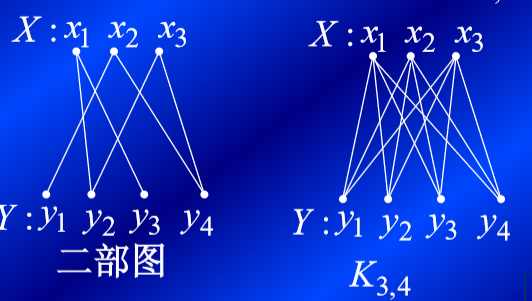起点和终点重合的道路称为闭通路

### 图与网络的数据结构

#### 邻接矩阵

0不相邻，1相邻

1234567表示顶点，1表示相通，0表示不通；

$w~ij~$$=\cases{权值&当Vi 与Vj 之间有边时\cr 0&当i=j时\cr∞&当Vi与Vj 之间无边时\cr} 有向图的邻接矩阵 A=（W~ij~）n×n aij==\cases{1&若（vi,vj）∈E\cr 0&若（vi,vj）∉E\cr} 无向图的邻接矩阵 A=（W~ij~）n×n a~ij~$$=\cases{1&$当Vi 与Vj 相邻$\cr 0&$当Vi与Vj 不相邻$\cr}$

#### 最短路径

MATLAB命令

https://www.cnblogs.com/markReaper/p/8454817.html

kruskal算法

https://mengzelev.github.io/2018/11/28/matchings/

https://zh.wikipedia.org/wiki/%E5%8C%B9%E9%85%8D_(%E5%9B%BE%E8%AE%BA)

https://www.renfei.org/blog/bipartite-matching.html

<最大匹配，匹配，理想匹配>

## 模糊聚类分析和模式识别

### 聚类分析

• 基于模糊函数的聚类分析方法：模糊传递闭包法，直接聚类法，最大树法和编网法
• 基于目标函数的聚类分析方法：称为模糊C均值聚类算法

#### 如何聚类分析

• 数据标准化
• 建立数据矩阵

• 标准差标准化 (使数据正规化，在$[0,1]$之间)

​ 极差正规化，极差标准化，最大值规格化

#### 聚类

• 自反性（$r_{ij}=1$）
• 对称性（$r_{ij}=r_{ji}$）
• 传递性（$R\circ R \subseteq R$）

相似性度量的相关、相似系数矩阵满足自反性和对称性，但不一定满足传递性。

对于传递性,可先计算$R○R(记作R^2)$,然后看其是否满足传递性。若不满足,经过$R○R=R^2, R^2○R^2=R^4 …$运 算,可将$R$改造成满足传递性的模糊等价矩阵;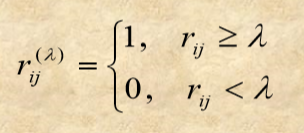• 对于不同的$λ∈[0,1]$，可得不同的分类方案，从而 形成一种动态聚类图。这对全面了解对象的分类情 况是比较形象和直观的。但有的实际问题需要选择 某个阀值λ，确定一个具体的分类，这就是确定阀 值λ的问题。

<动态聚类图>

### 模式识别

• 隶属度

• 模糊向量和内外积

0≤ai≤1(i=1,2,…,n),则称向量a=(a**1,**a2,…,a**n)为模糊向量。设ab**是模糊向量，则分别称：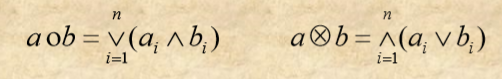​ 为向量ab内积和外积。符号$∧$和$∨$分别表示两个元素取小和取大。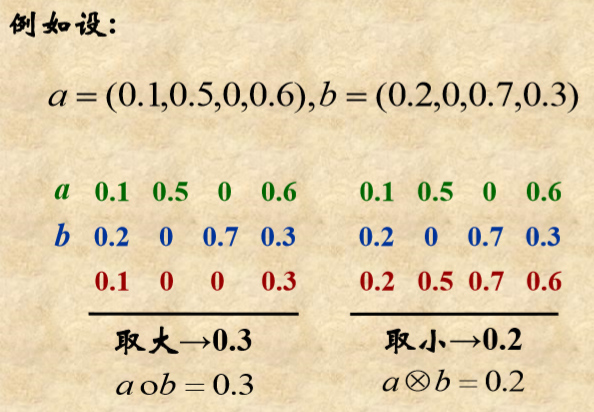• 最大隶属度原则

• 原则1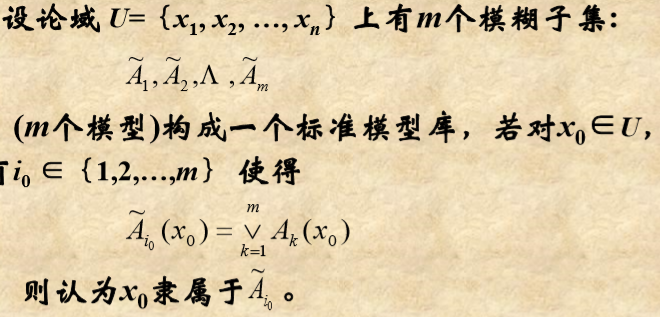• 原则2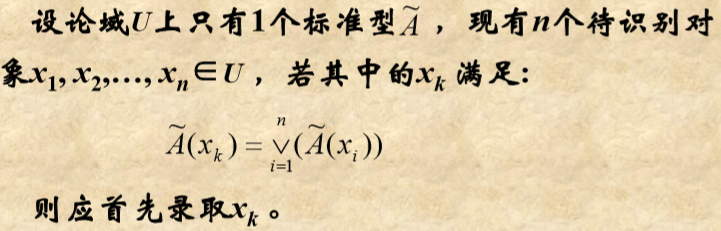• 贴近度

贴近度是描述模糊集之间彼此靠近程度的指标,是我国学者汪培庄教授提出的,由于研究的问题不同,贴近度也有不同的定义形式,它的一般定义为:

AB是论域$U$上的两个模糊子集，则称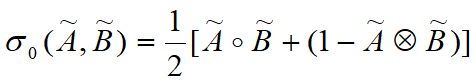A与B的贴近度

• 择近原则

## 时间序列

• $X_t=X_{t-1}+\delta_t$

• $X_t=Z_t\times\delta_t, Z_t$~N(0,1)